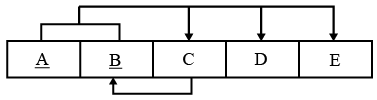# ISRO Scientist or Engineer Computer Science 2014

Instructions

For the following questions answer them individually

Question 61

# A mechanism or technology used in Ethernet by which two connected devices choose commontransmission parameters such as speed, duplex mode and flow control is calledQuestion 62

# Consider the following sorting algorithms.I. QuicksortII. HeapsortIiI. MergesortWhich of them perform in least time in the worst case?Question 63

# Consider the following tableThe table is in which normal form?Question 64

# Consider a 13 element hash table for which f(key) = key mod 13 is used with integer keys. Assuming linear probing is used for collision resolution, at which location would the key 103 be inserted,if the keys 661, 182, 24 and 103 are inserted in that order?Question 65

# A cube of side 1 unit is placed in such a waythatthe origin coincides with one of its top vertices and the three axes run along three of its edges. What are the co-ordinates of the vertex which is diagonally opposite to the vertex whose coordinates are (1, 0, 1)?Question 66

# Consider a system where each file is associated with a 16-bit number. For each file, each user should havethe read and write capability. How much memory is needed to store each user’s access data?Question 67

# What is the time complexity for the following C module? Assume that n > 0;int module(int n){ if( n == 1) return 1; else return (n + module(n-1));}Question 68

# What is the minimum numberof resources required to ensure that deadlock will never occur, if there are currently three processes P1, P2, and Ps running in a system whose maximum demand for the resources of same type are 3, 4 and 5 respectively.Question 69

# For a software project, the spiral model was employed. When will the spiral stop?Question 70

# Dirty bit is used to indicate which of the following?OR